## ML Aggarwal Class 10 Solutions for ICSE Maths Chapter 16 Constructions Chapter Test

These Solutions are part of ML Aggarwal Class 10 Solutions for ICSE Maths. Here we have given ML Aggarwal Class 10 Solutions for ICSE Maths Chapter 16 Constructions Chapter Test

More Exercises

Question 1.
Draw a circle of radius 3 cm. Mark its centre as C and mark a point P such that CP = 7 cm. Using ruler and compasses only, Construct two tangents from P to the circle.
Solution:
Steps of Construction :

1. Draw a circle with centre C and radius 3 cm.
2. Mark a point P such that CP = 7 cm.
3. With CP as diameter, draw a circle intersecting the given circle at T and S.
4. Join PT and PS.
5. Draw a tangent at Q to the circle given. Which intersects PT at D.
6. Draw the angle bisector of ∠PDQ intersecting CP at E.
7. With centre E and radius EQ, draw a circle.
It will touch the tangent T and PS and the given circle at Q.
This is the required circle.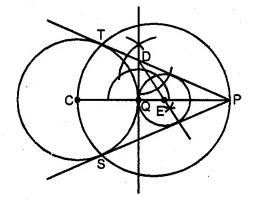Question 2.
Draw a line AQ = 7 cm. Mark a point P on AQ such that AP = 4 cm. Using ruler and compasses only, construct :
(i) a circle with AP as diameter.
(ii) two tangents to the above circle from the point Q.
Solution:
Steps of construction :

1. Draw a line segment AQ = 7 cm.
2. From AQ,cut off AP = 4cm
3. With AP as diameter draw a circle with centre O.
4. Draw bisector of OQ which intersect OQ at M.
5. With centre M and draw a circle with radius MQ
which intersects the first circle at T and S.
6. Join QT and QS.
QT and QS are the tangents to the first circle.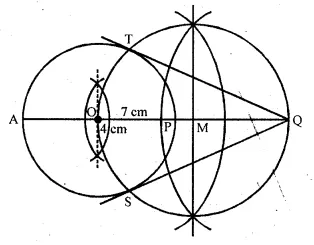Question 3.
Using ruler and compasses only, construct a triangle ABC having given c = 6 cm, b = 1 cm and ∠A = 30°. Measure side a. Draw carefully the circumcircle of the triangle.
Solution:
Steps of Construction :

1. Draw a line segment AC = 7 cm.
2. At C, draw a ray CX making an angle of 30°
3. With centre A and radius 6 cm draw an arc
which intersects the ray CX at B.
4. Join BA.
5. Draw perpendicular bisectors of AB and AC intersecting each other at O.
6. With centre O and radius OA or OB or OC,
draw a circle which will pass through A, B and C.
This is the required circumcircle of ∆ABC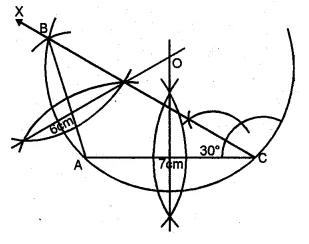Question 4.
Using ruler and compasses only, construct an equilateral triangle of height 4 cm and draw its circumcircle.
Solution:
Steps of Construction :

1. Draw a line XY and take a point D on it.
2. At D, draw perpendicular and cut off DA = 4 cm.
3. From A, draw rays making an angle of 30°
on each side of AD meeting the line XY at B and C.
4. Now draw perpendicular bisector of AC intersecting AD at O.
5. With centre O and radius OA or OB or OC
draw a circle which will pass through A, B and C.
This is the required circumcircle of ∆ABC.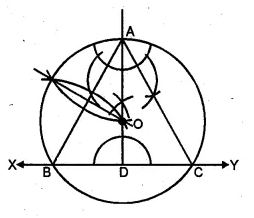Question 5.
Using ruler and compasses only :
(i) Construct a triangle ABC with the following data: BC = 7 cm, AB = 5 cm and ∠ABC = 45°.
(ii) Draw the inscribed circle to ∆ABC drawn in part (i).
Solution:
Steps of construction :

1. Draw a line segment BC = 7 cm.
2. At B, draw a ray BX making an angle of 45° and cut off BA = 5 cm.
3. Join AC.
4. Draw the angle bisectors of ∠B and ∠C intersecting each other at I.
5. From I, draw a perpendicular ID on BC.
6. With centre, I and radius ID, draw a circle
which touches the sides of ∆ABC at D, E and F respectively.
This is the required inscribed circle.Question 6.
Draw a triangle ABC, given that BC = 4cm, ∠C = 75° and that radius of the circumcircle of ∆ABC is 3 cm.
Solution:
Steps of Construction:

1. Draw a line segment BC = 4 cm
2. Draw the perpendicular bisector of BC.
3. From B draw an arc of 3 cm radius which intersects the perpendicular bisector at O.
4. Draw a ray CX making art angle of 75°
5. With centre O and radius 3 cm draw a circle which intersects the ray CX at A.
6. Join AB.
∆ABC is the required triangleQuestion 7.
Draw a regular hexagon of side 3.5 cm construct its circumcircle and measure its radius.
Solution:
Steps of construction:

1. Draw a regular hexagon ABCDEF whose each side is 3.5 cm.
2. Draw the perpendicular bisector of AB and BC
which intersect each other at O.
3. Join OA and OB.
4. With centre O and radius OA or OB, draw a circle
which passes through A, B, C, D, E and P.
Then this is the required circumcircle.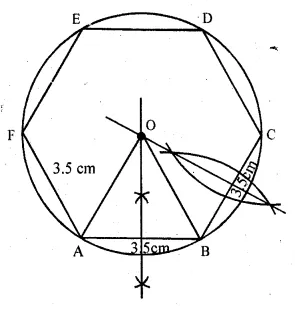Question 8.
Construct a triangle ABC with the following data: AB = 5 cm, BC = 6 cm and ∠ABC = 90°.
(i) Find a point P which is equidistant from B and C and is 5 cm from A. How many such points are there ?
(ii) Construct a circle touching the sides AB and BC, and whose centre is equidistant from B and C.
Solution:
Steps of Construction :

1. Draw a line segment BC = 6 cm.
2. At B, draw a ray BX making an angle of 90° and cut off BA = 5 cm.
3. Join AC.
4. Draw the perpendicular bisector of BC.
5. From A with 5 cm radius draw arc which intersects the perpendicular bisector of BC at P and P’.
There are two points.
6. Draw the angle bisectors of ∠B and ∠C intersecting at 0.
7. From O, draw OD ⊥ BC.
8. With centre O and radius OD, draw a circle which will touch the sides AB and BC.
This is the required circle.Hope given ML Aggarwal Class 10 Solutions for ICSE Maths Chapter 16 Constructions Chapter Test are helpful to complete your math homework.

If you have any doubts, please comment below. Learn Insta try to provide online math tutoring for you.